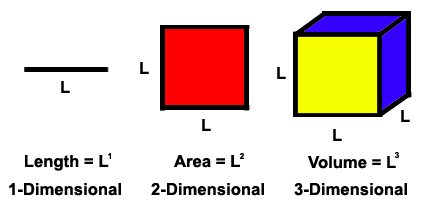HandsOn 6 - 1-, 2-, & 3-Dimensions

Fill in a copy of Table 2.4. Now plot the entries in this table on a fresh copy of the log-log graph paper. Along the horizontal axis plot the number of segments and, on the vertical axis, the entries in columns 2, 3, and 4. Draw a line through each set of points and verify that a line, square, and cube are 1-, 2-, and 3-dimensional, respectively.

Table 2.4: Verifying dimensions of a line, a square, and a cube.
 l Number of segments Number of squares Number of cubes of length d needed of side d needed of edge d needed to span line to cover square to fill cube of length L = 100 of area L2 = 10,000 of volume L3 = 106 1 2 5 10 20 50 100Figure 2.6: Examples of objects with 1, 2, and 3 dimensions.

Note that in Figure 2.6 the dimension is the exponent in the equation for length or area or volume, as a function of L.

Previous: 2.2 - Dimension of "Non-Fractals"
Next: HandsOn 7 - Circle Measurement of Dimension